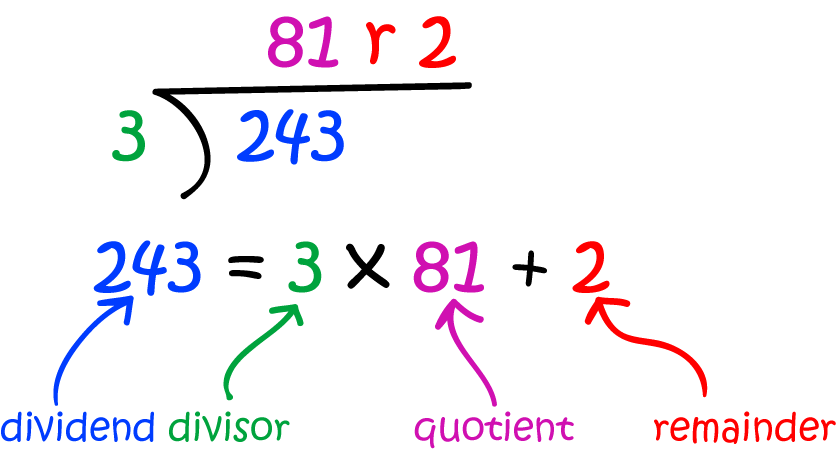# Definition of Quotient-Remainder TheoremThe quotient-remainder theorem is a result about arithmetic that tells us when we divide an integer $n$ by a positive integer, $d$, you can find unique integers $q$ (the quotient) and $r$ (the remainder) such that $n = dq + r$ and $0 \leq r For example, when \(n = 17$ and $d = 5$, $17 = 5 \times 3 + 2$. So, the quotient is $q = 3$ and the remainder is $r = 2$.

In the example on the left, $q = 81$ and $r = 2$.

The quotient-remainder theorem is what makes our algorithm for long division work.

### Description

The aim of this dictionary is to provide definitions to common mathematical terms. Students learn a new math skill every week at school, sometimes just before they start a new skill, if they want to look at what a specific term means, this is where this dictionary will become handy and a go-to guide for a student.

### Audience

Year 1 to Year 12 students

### Learning Objectives

Learn common math terms starting with letter Q

Author: Subject Coach
You must be logged in as Student to ask a Question.